# USE BASIC EXCEL FORMULAS – FAST AND EASY

Stop slaving over monotonous spreadsheets! Some people shy away from formulas, but they can save you hours of undue labour. You don’t need to be an Excel expert or a maths whiz to use them. Start using basic Excel formulas and gain these benefits:

• Less time wasted on repetitive work
• Reduced risk of calculation errors
• See clear results at a quick glance
• Capture data entry errors more easily
• Analyse your data more clearly

Formulas help you dive deep into your data and discover results you mightn’t have been aware of.

## What Are Basic Excel Formulas?

In Microsoft Excel, formulas are used to calculate the value of specific cells. For example, if you want to add rows A1 to A3, you would enter a formula in A4 that will add these values and present the total.## What Are Functions?

These are predetermined formulas. Using functions in Excel can save you time creating your own formulas. For example, using ‘Autosum’ to add up your cells: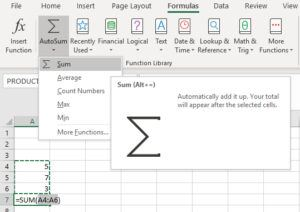## How to Add Basic Excel Formulas

There are various ways you can insert formulas into an Excel spreadsheet:

### Manually

Select a cell and type it in yourself. Whenever you use the equals symbol (=) Excel will recognise it as a formula. As you type, the formula will appear in the top formula bar.

1. Type equals (=) and your desired function.
2. Excel will give a pop-up of suggestions. To choose one, double-click on it or press the tab.
3. Using your mouse, select the cells you want to calculate.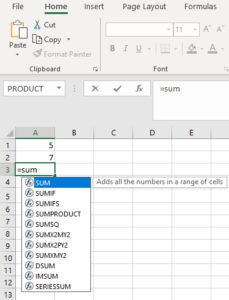### Insert Function

There’s a vast amount of formulas available to you. The Function Wizard helps determine which formula is best for your task. You can access this via ‘Insert Function.’

Go to Formulas tab → Insert Function.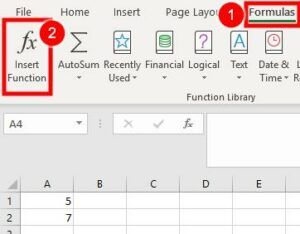This will display a pop-up box where you describe what you want to do. For example:

1. Type ‘multiply.’
2. The wizard suggests the formula ‘Product’, explaining this formula is used for multiplication.
3. Press ‘OK.’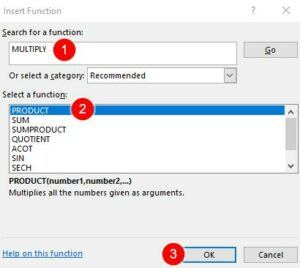1. Another box appears confirming details → check that it’s multiplying the correct cells → OK.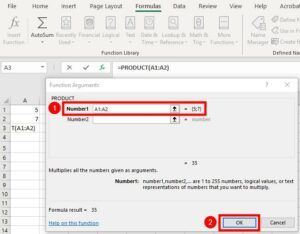The total will now appear in the cell you had typed the equals symbol into. The ‘Product’ formula is displayed in the Formula Bar for your reference.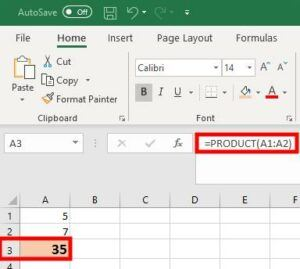Other ways to access the function wizard:

You can use a shortcut button located on the left-hand side of the formula bar.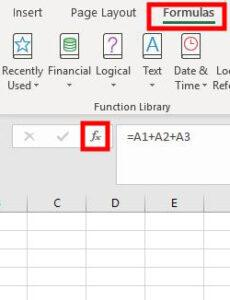Or you can use the ‘Name’ box, located on the far left of the formula bar:

1. Type the equals (=) symbol into a cell.
2. In the top left corner, press the box with a drop-down arrow.
3. Choose from the options or select ‘More Functions’ to access the Formula Wizard.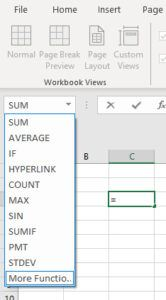### Autosum

This gives you 5 common functions with the option to search more.

1. Go to Formulas tab → Autosum → select from the drop-down menu.
2. For more options, select ‘more functions’ which will take you to the Function Wizard.

Aside from the Formula tab, you can find a shortcut to Autosum on the Home tab.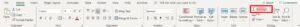### Select from Groups

You can find functions in different groups within the top ribbon.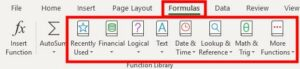For example:

1. Go to Formulas tab → press the ‘Financial’ or ‘Logical’ button → select from drop-down lists (or Insert Function to access the Function Wizard).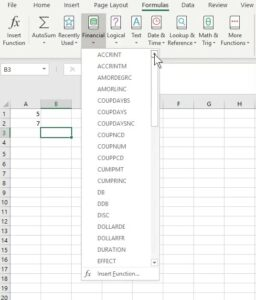### Select Recently Used

This tool is handy if you’re doing repetitive formulas. As the name implies, you can use it for recent formulas you’ve used.

Formulas tab → Recently Used → select from the drop-down list (or ‘Insert Function’ to access the Function Wizard).

Refer to the previous image.

## 10 Basic Excel Formulas & Functions

=A1+A2+A3

OR

=SUM(A1:A3)

You can include other functions within Sum.

For example: add cells A1 and A2 then multiply by A3. Using Parentheses () will ensure Excel calculates it in the order you want.

=SUM(A1+A2)*A3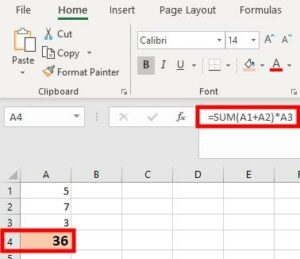See Video for another example.

### 2. Subtract

Subtract numbers in your selected cells.

=A1-A2-A3

And you can include negative numbers when calculating a group total.

=SUM(A1:A4)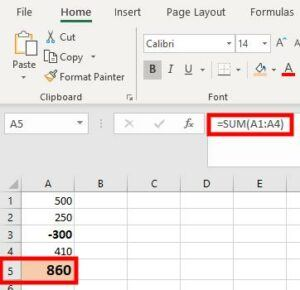### 3. Multiplication/Product

=A1*A2*A3

OR

=PRODUCT(A1:A3)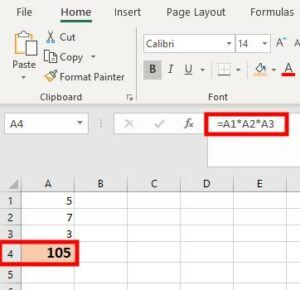### 4. Division/Quotient

=A1/A2

OR

=QUOTIENT(A1,A2)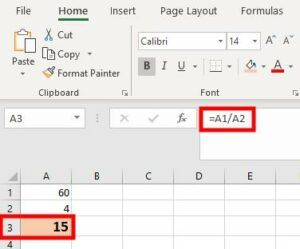### 5. Average

Works out the average of your range of cells.

=SUM(A1:A5)/5

OR

=AVERAGE(A1:A5)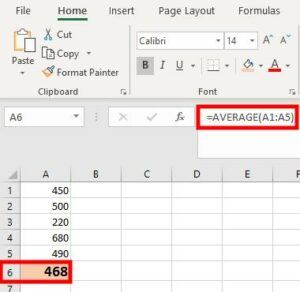### 6. If

This is used to make comparisons. Using greater (>) and less than (<) symbols can create a ‘true’ or ‘false’ result. For example, Expenses is less than Sales, therefore: =IFB1>B2   shows as ‘false’

=IFB1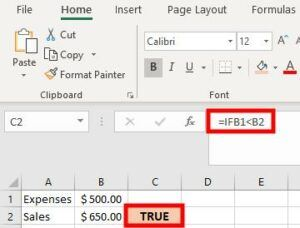You can manipulate it to show your desired wording. For example, label results as profit or loss:

If expenses are less than sales, then add “profit” to the formula.

=IF(B1<B2,”PROFIT”)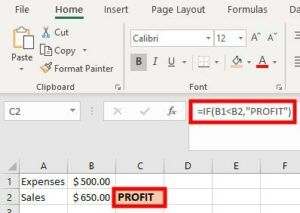### 7. Count

If you have a mixture of numbers and text, this function counts the number of cells with numerical data.

=COUNT(A1:A5)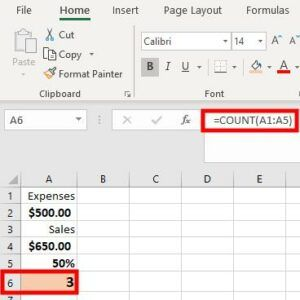### 8. Counta

This counts the total number of cells with values, including numerals and text. But it doesn’t include blank cells.

=COUNTA(B2:D5)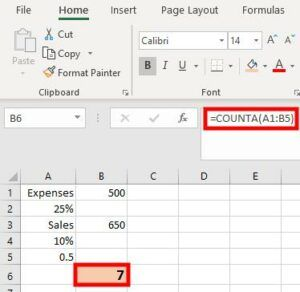### 9. Min & Max

These find the minimum or maximum number from a group of numerical data.

=MIN(B1:B5)

=MAX(B1:B5)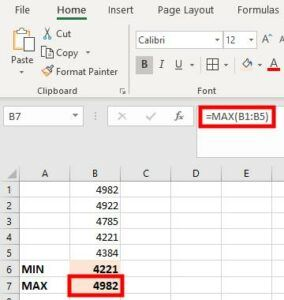### 10. Square Root

To calculate the square root of a number, use the SQRT function. This can be accessed a few ways, such as ‘Math & Trig’ group or ‘Insert Function.’

=SQRT(A1)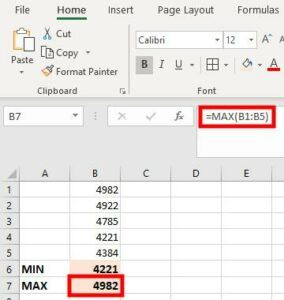You can view a detailed list of excel functions here.

## How to Edit Basic Excel Formulas

### Edit

You can do this manually. Double click on the cell and edit directly. Or click once on the cell and edit in the top formula bar.

Or you can use the ‘Autosum’ or ‘Insert Function’ tools:

1. Select the cell with a formula (in this case ‘sum’).
2. In the Autosum menu, select a new function (e.g. ‘Average’).
3. Press enter and this will change your formula.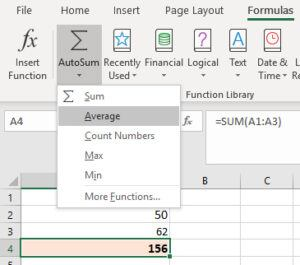### Duplicate

1. Select the cell.
2. Click on the handle in the bottom right corner and drag it down to the cell below.
3. Excel will copy the formula and automatically adjust the cells.

Or you can copy and paste the formula:

1. Select the cell to copy.
2. Press Ctrl C or right-click ‘Copy.’
3. Click on the cell below.
4. Press Ctrl V or right-click ‘Paste.’

See Video for demonstrations.

### Trim

To avoid formula errors, you can use a trim tool to eliminate empty spaces within a cell.

=TRIM(H21)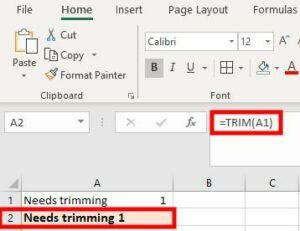>>View videos for more examples<<

## Basic Excel Formulas Make it Easier!

These are just some of the ways that formulas can save you a lot of time. You can speed up your progress by using Macros for repetitive formulas.

Microsoft Excel provides ample tools to make it easy to use. The more you use them, the easier it is to understand and expand on them. Use formulas to set up regular workbooks for rosters, itemising, stocktake, account reconciliation and so much more.

Got any tips on how you use formulas in the office? Share your ideas below!### 8 thoughts on “USE BASIC EXCEL FORMULAS – FAST AND EASY”

1.Cool. How do I do percentages?

•You can use the equation ‘select number divide total’ then press the % button. The % button is located in the top ribbon of the home tab (after ‘merge and center’).

Example:

cell B1 (50) / A1 (100) = 0.5
Press the percentage key which converts it to 50%

Keyboard shortcut: Ctrl+shift+%

Hope this helps.

•Cheers

2.A great basic introduction. Would love to put this post on my list of items to share out. May I please have your OK? Cheers Stacey

•Hi Stacey, please do share with anyone that’ll find it helpful. I appreciate it, thanks.

3.Done and posted on my site. Won’t share the link here but thank you so much and you saved me a lot of time as I was thinking of something like this for a while. Hope my attribution was suitable enough? Appreciated! I have a solid background in Excel and at times I have problems writing about the basics.

••